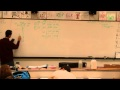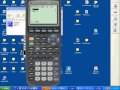### Study for the Chapter 4 Test

 Resources PreQuiz 4.1 | PreQuiz 9.1 Page 1 – Elevator problem.a. Acceleration with a certain tension - 2:19 Vertical Accel Part 1b. tension with an upward acceleration - Vertical Acceleration Example 1 - 2:38c. tension with a downward acceleration - 3:26 Vertical Accel Part 2 d. tension and acceleration with suvat - Vertical Accel + suvat Example 1e. tension and acceleration with suvat - Vertical Accel + suvat Example 4  Study:Net force: 4, 10, 11Prequiz 4.1 #4 Page 2 – Box sliding across the floor with frictiona+b. Calculate weight and kinetic friction.  Label forces as the box is dragged at a constant speed along the surface. - Read section 4-6, 4-8, especially look at figure 4-27- HTPIB04D More Than Once Force (I draw the picture you need to draw in the first 3:00) and Calculating Friction Example 1c. Acceleration with an applied force - Friction and Accel Example 1d. External force to get a certain acceleration - Friction and Accel Example 3e. External force with suvat - Friction and Accel Example 4Study:Net Force: 3, 5, 7, 13Prequiz 4.1 #3More things to study: Page 3 – Inclined plane with frictiona. Calculate Parallel, and Perpendicular gravity components along with kinetic and maximum static friction - 5:30 Solving Inclined Planes A (The first part)b. Will the block remain on the plane if it is at rest? Justify.  - 5:30 Solving Inclined Planes A (The last bit)c. Acceleration freely sliding down the plane. - 2:43 Solving Inclined Planes Bd. Acceleration with an given applied force -  Inclined Planes Example 6e. Force given an acceleration - Inclined Planes Example 2 Study:Inclined Planes: 1b, 2e, 2d, 4c, 3bMore things to study: Page 4 – Staticsa-b: Calculate the equilibrant - Solving for the EquilibrantMore Examples:c. Vertical equilibrium for a box on a plane with a string supporting some of its weight - read section 4-6 in the book. Especially pay attention to figure 4-15, and look at example 4-6(b and c) on page 82d-e: Two unknowns with matrices - force equilibrium9:54IBI 04-06-01 Prequiz 9.12:18PQ9 1 Matrices Study: